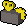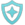## FANDOM

44,133 Pages

Not to be confused with Devotion.
For the improved perk, see Enhanced devoted.This article has a calculator here.Calculators determine experience and costs based on real-time prices from the Grand Exchange Market Watch.
 Description?3% chance per rank on being hit that protection prayers will work at 100% (or 75% in PvP) for 3 seconds. (Update) Armour 3 74

Devoted is an Invention perk that has a chance each hit of replicating the Devotion ability for 3 seconds. This effect does not put the ability itself on cooldown. Devoted has an effective level requirement of 74 as the materials required can only be unlocked at that level; however, enhanced devoted can be utilised at any Invention level for the cost of 2 perk slots. It can be created in armour gizmos.

Any type of damage, including typeless damage and self-inflicting damage, can activate the perk. The hit that activates Devoted will also be affected by the perk's effect. Devoted will play the same animation as Devotion whenever it activates, but uses its own icon in the buffs/debuffs interface. In player-versus-player situations the icon does not appear.

Devoted will be superseded by enhanced devoted if both are active.

Activation chance
Rank Chance (level 20)
1 3% (3.3%)
2 6% (6.6%)
3 9% (9.9%)

## Calculations

For PvM:

• The average ratio of damage taken, $r_{avg}$, from Devoted to that of without Devoted is
$r_{avg} = \frac{\sum\limits_{n=0}^{\infty}\left(1-p_{R}\right)^{n}p_{R}\left[\left(\sum\limits_{i=1}^{n}d^{(0)}_{n,i}\right) + \left\lceil{\frac{t_{d}}{t_{AS}}}\right\rceil \right]}{\sum\limits_{n=0}^{\infty}\left(1-p_{R}\right)^{n}p_{R}\left[\sum\limits_{i=1}^{n}d^{(0)}_{n,i} + \sum\limits_{i=1}^{\left\lceil{\frac{t_{d}}{t_{AS}}}\right\rceil}d^{(1)}_{n,i} \right]}$
• The average damage reduction is then, after some rearranging,
$1-r_{avg} = \frac{\sum\limits_{n=0}^{\infty}\left(1-p_{R}\right)^{n}p_{R}\sum\limits_{i=1}^{\left\lceil{\frac{t_{d}}{t_{AS}}}\right\rceil}\left(d^{(1)}_{n,i} - 1\right)}{\sum\limits_{n=0}^{\infty}\left(1-p_{R}\right)^{n}p_{R}\left[\sum\limits_{i=1}^{n}d^{(0)}_{n,i} + \sum\limits_{i=1}^{\left\lceil{\frac{t_{d}}{t_{AS}}}\right\rceil}d^{(1)}_{n,i} \right]}$

For PvP :

• The average ratio of damage taken, $r_{avg}$, from Devoted to that of without Devoted is
$r_{avg} = \frac{\sum\limits_{n=0}^{\infty}\left(1-p_{R}\right)^{n}p_{R}\left[\sum\limits_{i=1}^{n}d^{(0)}_{n,i} + \sum\limits_{i=1}^{\left\lceil{\frac{t_{d}}{t_{AS}}}\right\rceil} \left\lfloor{.5 \times d^{(1)}_{n,i}}\right\rfloor \right]}{\sum\limits_{n=0}^{\infty}\left(1-p_{R}\right)^{n}p_{R}\left[\sum\limits_{i=1}^{n}d^{(0)}_{n,i} + \sum\limits_{i=1}^{\left\lceil{\frac{t_{d}}{t_{AS}}}\right\rceil}d^{(1)}_{n,i} \right]}$
• The average damage reduction is then, after some rearranging,
$1-r_{avg} = \frac{\sum\limits_{n=0}^{\infty}\left(1-p_{R}\right)^{n}p_{R}\sum\limits_{i=1}^{\left\lceil{\frac{t_{d}}{t_{AS}}}\right\rceil}\left(d^{(1)}_{n,i} - \left\lfloor{.5 \times d^{(1)}_{n,i}}\right\rfloor \right)}{\sum\limits_{n=0}^{\infty}\left(1-p_{R}\right)^{n}p_{R}\left[\sum\limits_{i=1}^{n}d^{(0)}_{n,i} + \sum\limits_{i=1}^{\left\lceil{\frac{t_{d}}{t_{AS}}}\right\rceil}d^{(1)}_{n,i} \right]}$
Notes
• A calculator for this is at the top of this page.
• Assumptions :
• The enemy is always attacking with the same style that matches the protect prayer (deflect curse) used.
• The enemy is always attacking at the same attack speed.
• $R$ is the rank of the Devoted perk.
• $p_{R}$ is the proc chance of Devoted to activate (if the perk is on level 20 gear, then this value is multiplied by 1.1).
• $\textstyle\sum_{n=0}^{\infty}\left(1-p\right)^{n}p = 1$ when $0 \leq p < 1$ or $\left|1 - p\right| < 1$. This represents summing over all possibilities where integer $n \in [0,\infty)$ represents the amount of hits prior to the proc of Devoted. The probability of Devoted proccing on hit ${n+1}$ is therefore $\left(1-p\right)^{n}p$.
• $t_{d}$ is the time that Devoted is active. This is taken to be 5 game ticks.
• $t_{AS}$ is the attack speed of the enemy in game ticks.
• $d^{(j)}_{n,i}$ is the $i^{th}$ random value uniformly sampled between the enemy's minimum hit and maximum hit. The $j$ describes different sets of hits. The sets are regenerated for every new value of $n$.
• $d^{(0)}_{n,i}$ has $n$ elements with integer $i \in [1,n]$. This is the damage taken before the perk procs.
• Clarification : This does mean that for $n=0$ that there are no elements in this set.
• $d^{(1)}_{n,i}$ has $\left\lceil{\frac{t_{d}}{t_{AS}}}\right\rceil$ elements with integer $i \in \left[1,\left\lceil{\frac{t_{d}}{t_{AS}}}\right\rceil\right]$. This is the damage taken during the time where Devoted procced.
• The set of values in $d^{(j)}_{n}$ for integer $j \in [0,1]$ is the same in both the numerator and denominator of the ratios for any given $n$.

Simplifications
• This can be simplified if the assumption is that every value of $d_{n,i}^{(j)}$ $\forall$ integer $i \in [1,n]$, integer $n \in [0,\infty)$, integer $j \in [0,1]$, is taken to be the same $\left(d_{n,i}^{(j)} \rightarrow d\right)$. In this scenario, there is no random element and the above $r_{avg}$ is then only dependent on the proc chance and the attack speed of the enemy. This simplification is increasingly accurate in the limit of large $d$. All examples are using large enough values of $d$ such that the values should not change to the first decimal place in percent. Two tables are provided below. The first covers all values of $t_{AS}$ for PvM situations. The second table is for PvP for all values of $t_{AS}$ (normal attack speed of player is $t_{AS} = 3$ in non-legacy mode , but all values of $t_{AS}$ have been provided to account for proccing on damage-over-time abilities, 4 tick auto attack, etc., as well as the varied attack speeds of weapons if using legacy combat).

Using these simplifications, the damage reduction reduces to

For PvM :

$1 - r_{avg} = \frac{\left\lceil{\frac{t_{d}}{t_{AS}}}\right\rceil p_{R}}{\left\lceil{\frac{t_{d}}{t_{AS}}}\right\rceil p_{R} + \left(1-p_{R}\right)}$

For PvP :

$1 - r_{avg} = \frac{.5 \left\lceil{\frac{t_{d}}{t_{AS}}}\right\rceil p_{R}}{\left\lceil{\frac{t_{d}}{t_{AS}}}\right\rceil p_{R} + \left(1-p_{R}\right)}$
PvM average damage reduction
Rank $t_{AS} = 1$ $t_{AS} = 2$ $t_{AS} = 3$ or $4$ $t_{AS} = 5+$
1 13.4% (14.6%) 8.5% (9.3%) 5.8% (6.4%) 3.0% (3.3%)
2 24.2% (26.1%) 16.1% (17.5%) 11.3% (12.4%) 6.0% (6.6%)
3 33.1% (35.5%) 22.9% (24.8%) 16.5% (18.0%) 9.0% (9.9%)
• All numbers in parentheses refer to level 20 gear.
PvP average damage reduction
Rank $t_{AS} = 1$ $t_{AS} = 2$ $t_{AS} = 3$ or $4$ $t_{AS} = 5+$
1 6.7% (7.3%) 4.2% (4.6%) 2.9% (3.2%) 1.5% (1.6%)
2 12.1% (13.1%) 8.0% (8.7%) 5.7% (6.2%) 3.0% (3.3%)
3 16.5% (17.7%) 11.4% (12.4%) 8.3% (9.0%) 4.5% (5.0%)
• All numbers in parentheses refer to level 20 gear.

## Sources

MaterialRarityPerk ranks with X materials
12345